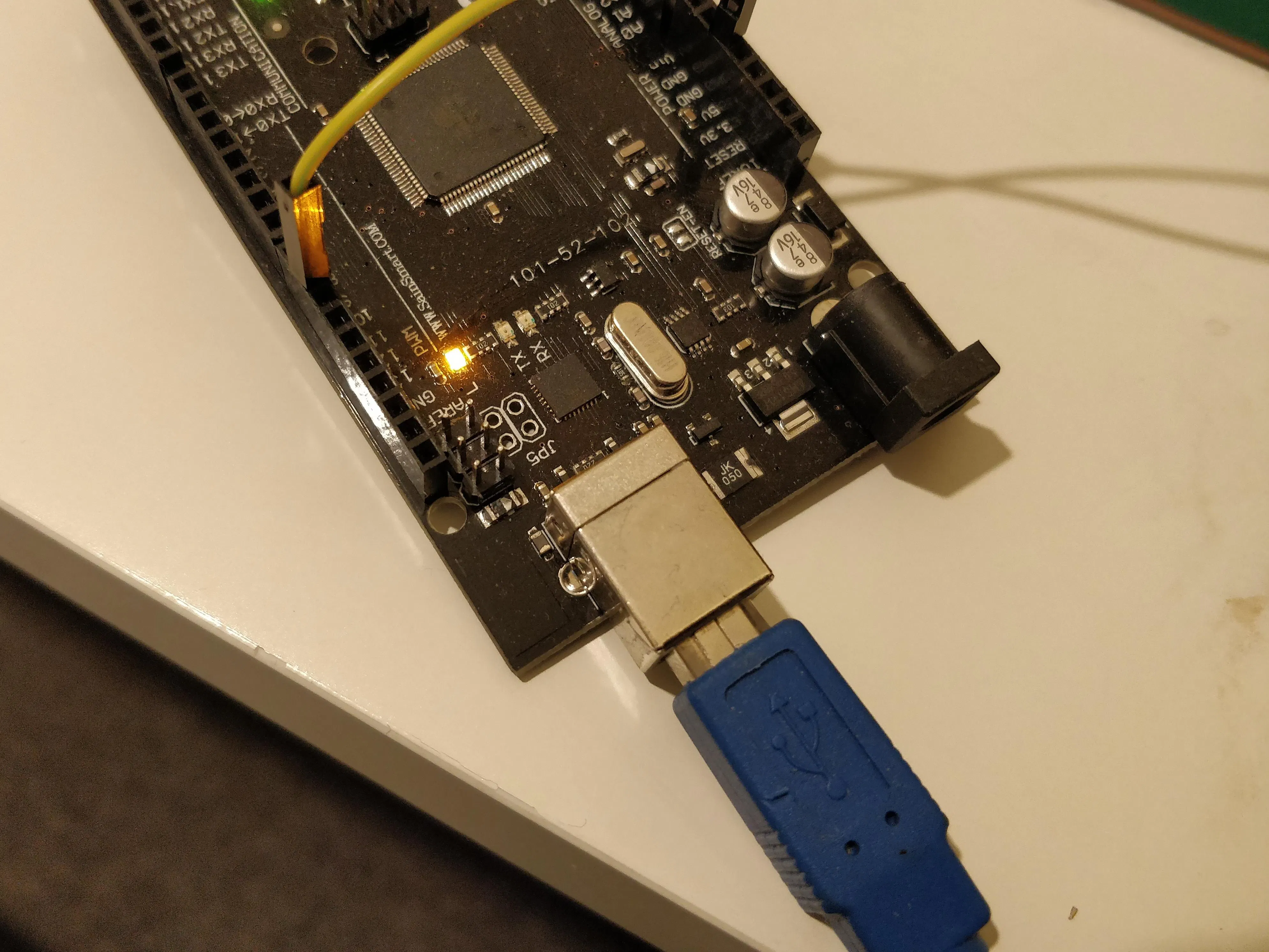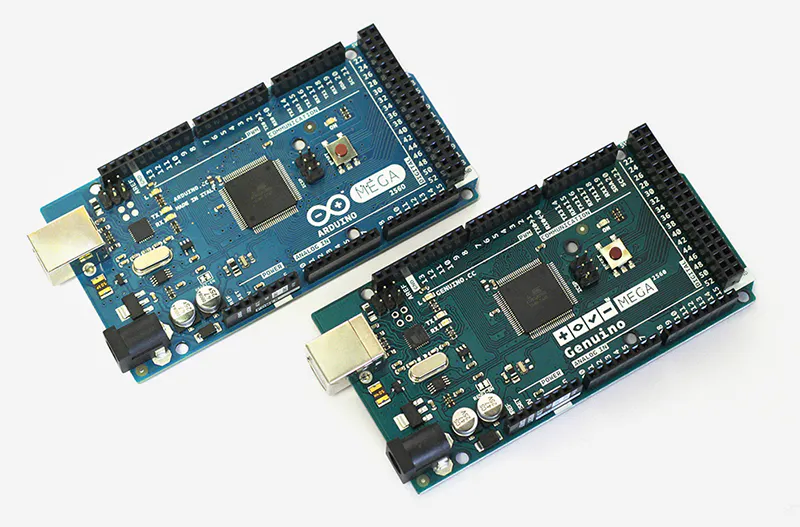# How to Get String Data from Computer via Serial Port © MIT

This project helps you to send strings from computer to your Arduino via serial port.

• 499 views
• 5 respects

## Components and suppliesArduino Mega 2560 & Genuino Mega 2560 You could use any other Arduino board. It just so happens that I have this one
×1

## Apps and online services

We will be using the Arduino language to collect string data from a Serial port. This will allow us to change specific values of the code whilst the data is running - for example, we can change the speed of a motor or the colour of an LED without having to adjust the code and/or restarting the program. This project will give you the code and explain what is happening in each line.

### The Code

Starting the code, we have the following lines:

``#define LF          0x0A char angle_str;int idx;``

'angle_str' is an array of maximum 10 characters used to store a string, and 'idx' will later be used as a positional pointer, tracking the current byte within the array. To use 'angle_str' as a c-string, we will need to end the string with NULL character (0x00), for more information, click here.

I will explain the LF later.

Before we go into the initial loop(), we must setup the serial port and the starting position within the serial buffer 'angle_str' by adding the following code:

``void setup() {    Serial.begin(9600);    Serial.print("start\r\n");    idx = 0;}``

If you have done a bit of work with Serial or even have just used Arduino, you will probably be familiar with Serial.begin(). In this case, Serial.begin(9600) means the data rate will be 9600 bits per second. The setting for serial port's speed on your computer must match the speed stated within Serial.begin() for it to work.

'idx' indicates where the byte received from serial port should be stored in the 'angle_str' array. Starting with 0 means we are saving it to the first element of the array.

In the loop() function, first, add the following:

``if (Serial.available() > 0) {``

The if() is used to check whether there is any data in the Serial buffer. If yes, then the next command inside the if() statement will be executed as following:

``angle_str[idx] = Serial.read();``

This code collects one byte from the serial buffer and stores it in the 'angle_str' array corresponding to 'idx' position. This happens each time you type a letter or number. In order to move on to the next element, 'idx' has to increase by one as we will see later.

``if (angle_str[idx] == LF) {``

Also, within that if() statement, we have another if() statement to check whether we have finished the string by pressing the Enter key. Usually, when we press Enter, the computer will send two characters, CR/LF. In ASCII, these values are 0x0D and 0x0A, respectively.

This statement checks if the character at the current position of 'angle_str' is 'LF' which is an abbreviation for line feed (makes the cursor move down a key), then we have received all the characters of the string.

Next statements are as following:

``Serial.print("Received new angle: ");angle_str[idx-1] = 0;Serial.println(angle_str);``

First, we re-print the string for debugging purposes. The second statement is to mark the end of the c-string with the character NULL, which is represented by 0. We used [idx-1] to allow us to position the character after the last byte of the c-string. The string is also printed for debugging.

After that, we must reset the initial position of the 'angle_str' array back to 0. However, we set it to -1 here because it will be increased later.

``idx = -1;``

If we did not hit the Enter key, the c-string continues and therefore, we must move 'idx' forward by one. This is why we have set it to -1 earlier.

``idx++; //``

To conclude, your code should look like this:

``#define LF          0x0A char angle_str; int idx; void setup() {    Serial.begin(9600);    Serial.print("start\r\n");    idx = 0;}void loop() {    if (Serial.available() > 0) {    angle_str[idx] = Serial.read();    if (angle_str[idx] == LF) {        Serial.print("Received new angle: ");        angle_str[idx-1] = 0;        Serial.println(angle_str);        idx = -1;        }    idx++;    }}``

## Code

##### Serial CodeArduino
```#define LF          0x0A

char angle_str;
int idx;

void setup() {
Serial.begin(9600);
Serial.print("start\r\n");

idx = 0;
}

void loop() {

if (Serial.available() > 0) {
if (angle_str[idx] == LF) {
angle_str[idx-1] = 0;
Serial.println(angle_str);
idx = -1;
}
idx++;
}
}
```

January 9, 2020

#### Members who respect this project

See similar projects
you might like

• 5,253 views
• 11 respects

• 12,450 views
• 31 respects

#### Serial it

Project tutorial by Tal O

• 1,121 views
• 0 respects

• 10,651 views
• 1 comment
• 21 respects

• 88,294 views